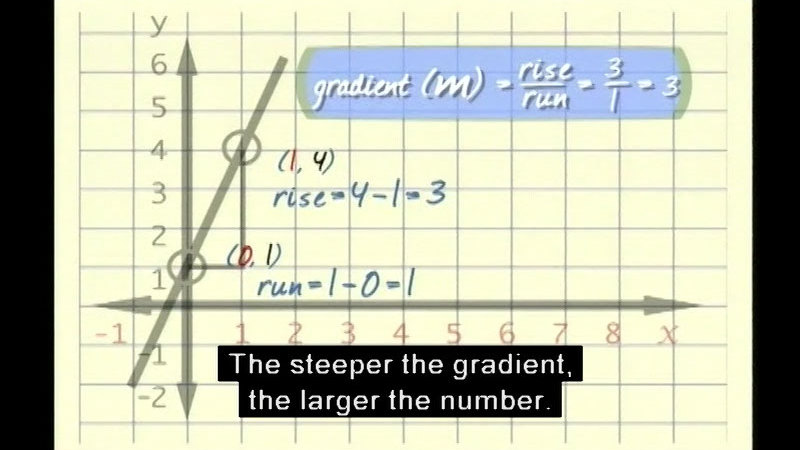# Linear Functions: An Introduction

21 minutes 16 seconds

Videos are generally available for preview to non-members as short clips. Limited full-length titles are also available. Log In to view the full length title.Linear functions are one of the most important and basic of functions studied in algebra. Shows the different forms of linear functions, tables, graphs, and equations. Explores the concept of the y-intercept and how to determine the slope of a line given any two points on a line. Outlines how to plot linear graphs from linear equations and explains how, with the gradient and y-intercept, it is possible to not only sketch the linear graph but also determine the linear equation for a line.

## Media Details

Runtime: 21 minutes 16 seconds